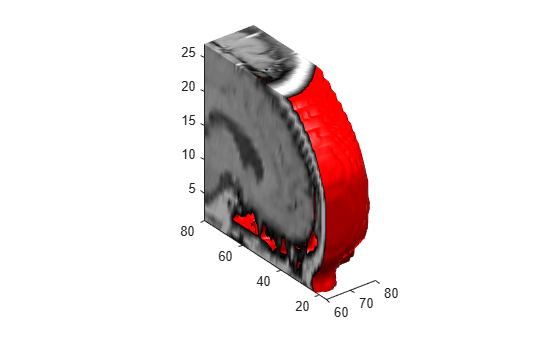Main Content

# subvolume

Extract subset of volume data set

## Syntax

```[Nx,Ny,Nz,Nv] = subvolume(X,Y,Z,V,limits) [Nx,Ny,Nz,Nv] = subvolume(V,limits) Nv = subvolume(...) ```

## Description

`[Nx,Ny,Nz,Nv] = subvolume(X,Y,Z,V,limits) ` extracts a subset of the volume data set `V` using the specified axis-aligned `limits`. ```limits = [xmin,xmax,ymin, ymax,zmin,zmax]``` (Any `NaN`s in the limits indicate that the volume should not be cropped along that axis.)

The arrays `X`, `Y`, and `Z` define the coordinates for the volume `V`. The subvolume is returned in `NV` and the coordinates of the subvolume are given in `NX`, `NY`, and `NZ`.

`[Nx,Ny,Nz,Nv] = subvolume(V,limits) ` assumes the arrays `X`, `Y`, and `Z` are defined as

```[X,Y,Z] = meshgrid(1:N,1:M,1:P) ```

where `[M,N,P] = size(V)`.

`Nv = subvolume(...) ` returns only the subvolume.

## Examples

collapse all

Load the `mri` data set, which is a collection of MRI slices of a human skull. Then:

• Squeeze the four-dimensional array into three dimensions and extract a subset using `subvolume`.

• Use a patch to display an isosurface of the skull outline.

• Add a second patch with interpolated face colors.

• Set the view of the object.

• Change the colormap to a grayscale colormap.

• Add lights to the right and left of the camera to illuminate the object.

```load mri D = squeeze(D); [x,y,z,D] = subvolume(D,[60,80,nan,80,nan,nan]); p1 = patch(isosurface(x,y,z,D, 5),... 'FaceColor','red','EdgeColor','none'); isonormals(x,y,z,D,p1); p2 = patch(isocaps(x,y,z,D, 5),... 'FaceColor','interp','EdgeColor','none'); view(3); axis tight; daspect([1 1 0.4]) colormap(gray(100)) camlight right; camlight left; lighting gouraud```## See Also

Introduced before R2006a

Download ebook